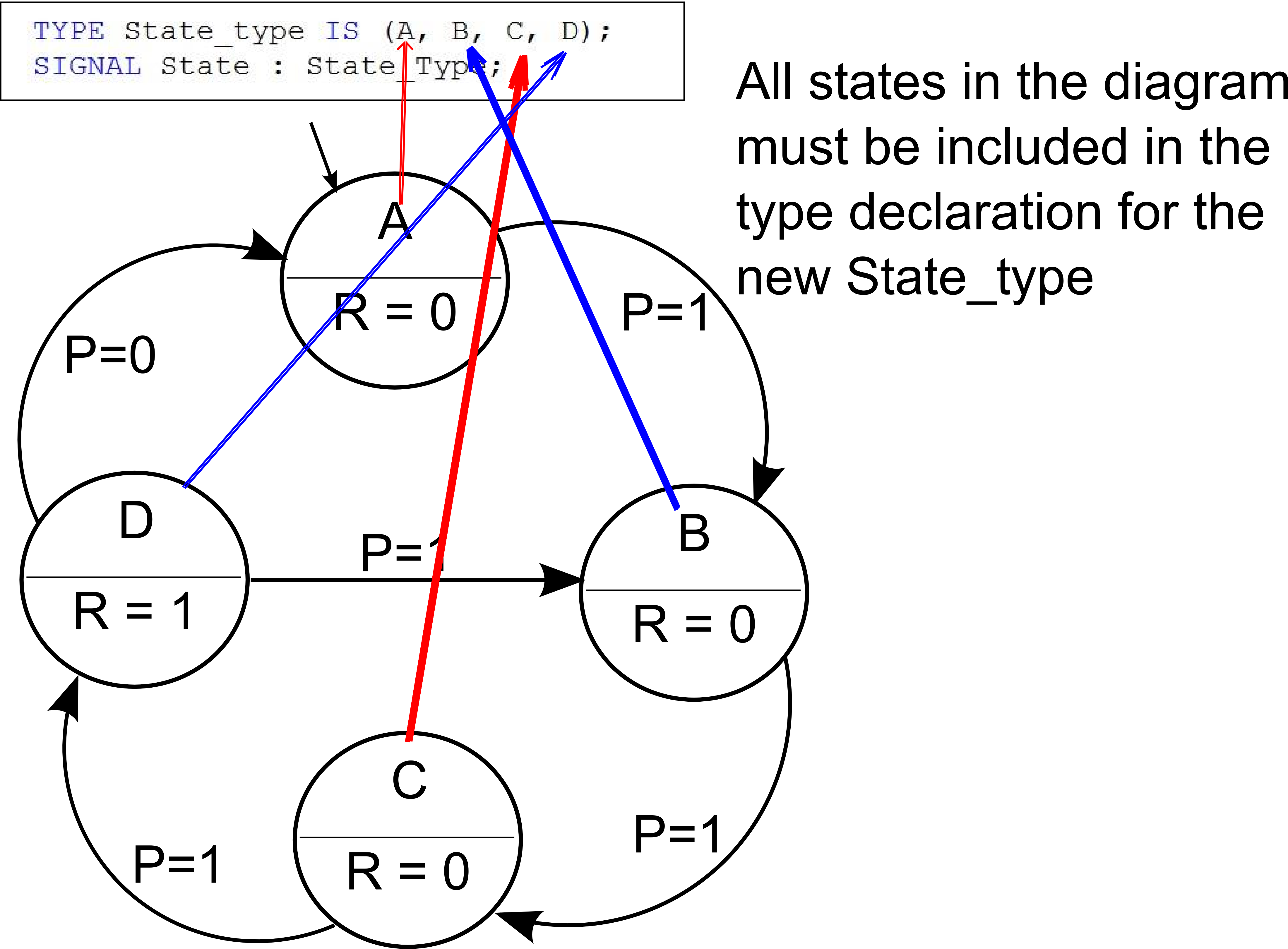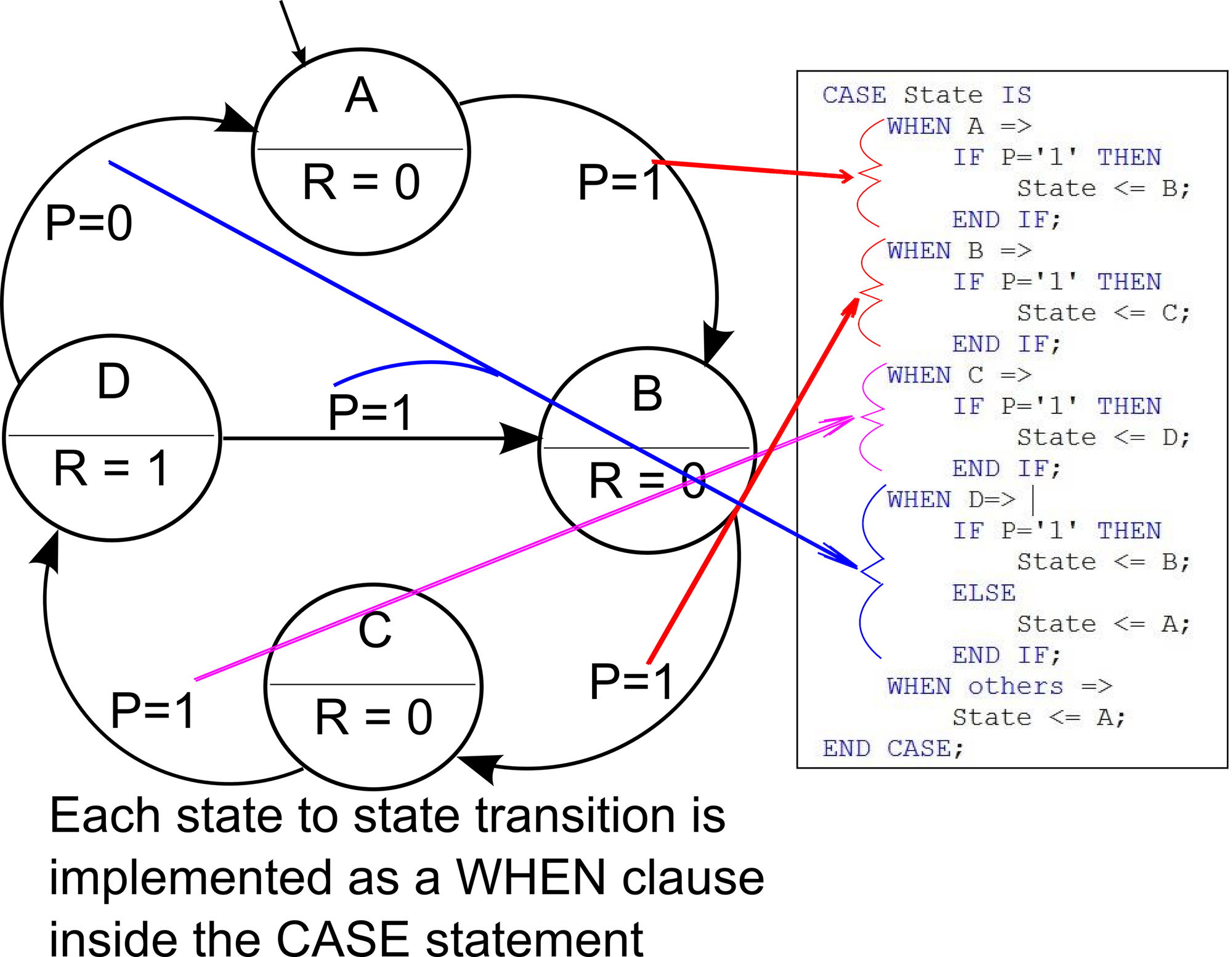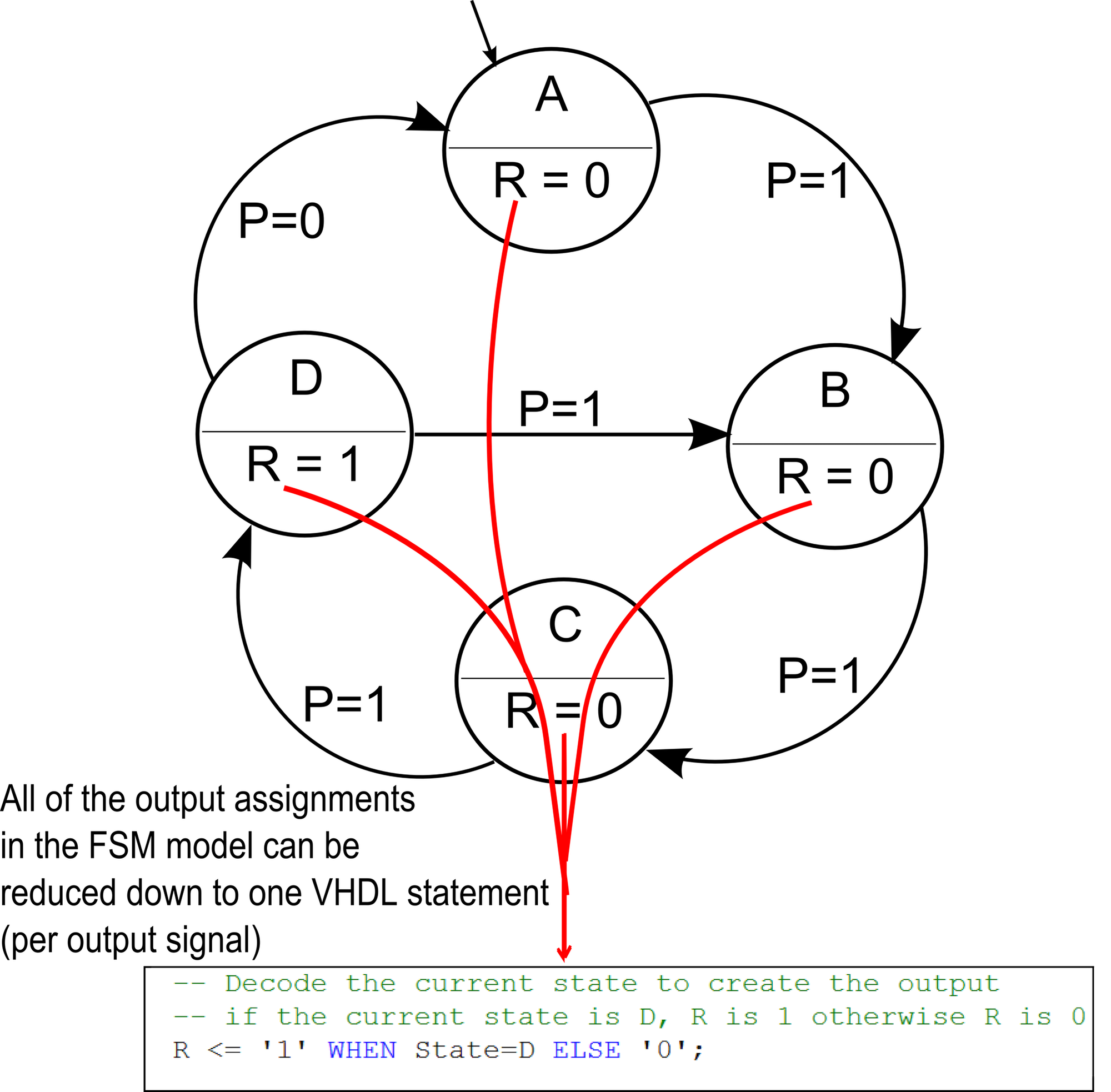Technical Article

# Implementing a Finite State Machine in VHDL

December 23, 2015 by David Williams

## Impress your friends and family by learning how to implement a finite state machine in VHDL

### A Bit of Background

In digital systems, there are two basic types of circuits. The first type are combinational logic circuits. In combinational logic circuits, the outputs depend solely on the inputs. Examples of combinational logic circuits include adders, encoders, and multiplexers. In adders, for example, the output is simply the sum of the inputs; it doesn't matter what any of the previous inputs or outputs were. The second type of digital logic circuits are sequential logic circuits. In sequential logic circuits, the outputs depend not only on the inputs, but also on the present state of system (i.e., the values of the outputs and any internal signals or variables). Sequential logic circuits range in complexity from simple counters that move from one state to another in a basic sequence (e.g., 0,1,2,3…0,1,2,3…) to very large scale circuits such as microprocessors with millions of different states or more.The focus of this article will be on the representation of sequential logic circuits as finite state machines and how to convert those finite state machines into the hardware description language VHDL.

Sequential logic systems are finite state machines (FSMs). As FSMs, they consist of a set of states, some inputs, some outputs, and a set of rules for moving from state to state. When doing digital system design, it is very common to begin by defining how the system works with a finite state machine model. This design step allows the designer to think about the design from a high-level point of view without having to think much about what kind of hardware the system will be implemented on or what design tools will be required to implement the design. Once the FSM is fully designed, if it is designed well, it is easy to write out the design in a hardware description language (such as Verilog or VHDL) for implementation on a digital IC (integrated circuit).

This article will go through the design process of creating a digital system by first defining a design problem, second, creating the computational model of the system as a finite state machine and third, translating the FSM into the hardware description language VHDL. (VHDL is actually a double acronym. VHDL stands for VHSIC Hardware Description Language and VHSIC stands for Very High Speed Integrated Circuit).

Readers should have some experience with digital circuits and ICs. They should also have a basic understanding of VHDL or at least have some experience reading structured computer code. Experience with computer code will help you recognize some of the structures and constructs of VHDL, but it should be noted that VHDL is not a programming language; it is a hardware description language (HDL). In other words, the statements that you write are going to create hardware (gates, flip flops etc.) in the system you are designing.

### The Finite State Machine

The system to be designed is a very simple one and its purpose is to introduce the idea of converting a FSM into VHDL. This FSM has four states: A, B, C, and D. The system has one input signal called P, and the value of P determines what state the system moves to next. The system changes state from A to B to C to D as long as the input P is high (1). If P is low, and the system is in state A, B, or C, the state is not changed. If the system is in state D, it changes to B if P is high and to A if P is low. The system also has an output called R which is 1 if in state D, otherwise it is a 0. Figure 1 is the diagram for the FSM, but first here are a few notes about this diagram:

• The circles represent the states
• Arrows between the circles represent the rules for changing from state to state. For example, in this system, the state machine moves from state A to state B if the input P is equal to 1 (otherwise it remains in state A)
• The information underneath the line in the circle represents the output value when in each state.
• The arrow coming from "nowhere" to the A indicates that A is the initial state.Figure 1. A Simple Finite State Machine

This fully defined state machine can very easily be converted into VHDL. It is important to remember that when writing the VHDL code, what you are doing is describing how you want the hardware (i.e., the digital gates) implemented. So, for example, when you define a set of states like A, B, C, and D in this system, those states are going to be represented by bits, and more specifically by the output of flip flops. In a system with four states, like this one,  it would be possible to represent those four states with 2 bits (2 flip flops). There are other ways that the states could be represented too.  One of those ways would be to use four bits, where each bit represents a state, but only one bit can be on at a time.  So A would be represented by 0001, B by 0010, C by 0100 and D by 1000. One of the good things about using a high level hardware description language is that you can often ignore this level of detail.

Figure 2 shows the general idea of the hardware circuitry that will be created when the VHDL code is synthesized to create the hardware.Figure 2. Block Diagram Representation of Logic Created for a State Machine

This diagram indicates that there is a set of n flip flops that represent the state. There is also some logic that uses the output of the flip flops and the inputs to the system to determine the next state. Finally, there is some logic that decodes the output values of the flip flops to create the m output signals.

Again, when using a HDL, you can often ignore this level of detail in your design. It is still important to understand what kind of circuitry is created by your HDL because there may come a time when you have to count and minimize the number logic gates in your design. With an understanding of what is created by your HDL statements you can then design to minimize gate creation.

### VHDL Implementation of Design

The first step in writing the VHDL for this FSM is to define the VHDL entity. The VHDL entity describes the external interface of the system you are designing which includes the inputs, the outputs, and the name of the entity. The general form of an entity looks like this:


ENTITY -entityName- is

PORT (-portName1- : -signalDirection- -type-;
-portName2- : -signalDirection- -type-;
---
-portName_n- : -signalDirection- -type-;
END -entityName-;

--Note: user defined entries are between the dashes


Using this template, the entity for the simple FSM can be created. The name of the entity will be SimpleFSM, the inputs are a clock signal, the reset signal and the P signal, and the output is the R signal. It should be mentioned that the clock signal is the periodic high-low signal that controls the timing to this synchronous system. Any synchronous system has one controlling clock signal that synchronizes all of the blocks in the system making them change at the same time.

Putting all of the information together gives a SimpleFSM entity that looks like this:

ENTITY SimpleFSM is
PORT (clock:    IN STD_LOGIC;
P:        IN STD_LOGIC;
reset:    IN STD_LOGIC;
R :       OUT STD_LOGIC);
END SimpleFSM;

One final note about the entity is that all the inputs and outputs are single bits and so can use the data type std_logic which is the standard type in VHDL for single bit signals.The next step is to define the functionality of the entity; this block of VHDL is called the architecture. The functionality that we are implementing is that of the state machine defined in figure 1. The example below shows the code that would be needed to implement the SimpleFSM. While this code is specific to the SimpleFSM, I will describe what each of the sections of the code do so that it will be an easy process to replace this code with code for your own state machine.


-- Architecture definition for the SimpleFSM entity
Architecture RTL of SimpleFSM is
TYPE State_type IS (A, B, C, D);  -- Define the states
SIGNAL State : State_Type;    -- Create a signal that uses
-- the different states
BEGIN
PROCESS (clock, reset)
BEGIN
If (reset = ‘1’) THEN            -- Upon reset, set the state to A
State <= A;

ELSIF rising_edge(clock) THEN    -- if there is a rising edge of the
-- clock, then do the stuff below

-- The CASE statement checks the value of the State variable,
-- and based on the value and any other control signals, changes
-- to a new state.
CASE State IS

-- If the current state is A and P is set to 1, then the
-- next state is B
WHEN A =>
IF P='1' THEN
State <= B;
END IF;

-- If the current state is B and P is set to 1, then the
-- next state is C
WHEN B =>
IF P='1' THEN
State <= C;
END IF;

-- If the current state is C and P is set to 1, then the
-- next state is D
WHEN C =>
IF P='1' THEN
State <= D;
END IF;

-- If the current state is D and P is set to 1, then the
-- next state is B.
-- If the current state is D and P is set to 0, then the
-- next state is A.
WHEN D=>
IF P='1' THEN
State <= B;
ELSE
State <= A;
END IF;
WHEN others =>
State <= A;
END CASE;
END IF;
END PROCESS;

-- Decode the current state to create the output
-- if the current state is D, R is 1 otherwise R is 0
R <= ‘1’ WHEN State=D ELSE ‘0’;
END rtl;

That is the entirety of the code needed for the state machine. Now let’s look at some of the details of the architecture code.

The architecture definition states:


Architecture RTL of SimpleFSM is


This statement is a standard one for a VHDL architecture and it basically states the level of abstraction that will be described in the architecture. RTL, which stands for register-transfer level, is a mid-level of abstraction.

Behavioural is the highest level of abstraction and when writing behavioural code you simply need to define the relationships between inputs and outputs without specifying anything about how those relationships will be implemented. Sometimes behavioural descriptions are too high level and cannot actually be synthesized into hardware. If you are doing a simulation and just need a block to behave in a certain way, then a behavioural model will be adequate.

Structural code is the lowest level of abstraction. When writing structural code, you describe how the low level structures (e.g., logic gates) connect together to give the system that you want. If you need precise control over the logic gates that will be created, a structural model is what you need.

RTL fits in the middle. It specifically describes the relationships between inputs and outputs be describing how data moves between registers in the hardware. RTL descriptions are implementable in hardware. For this particular example, it is not very important to understand the nuances of the architecture type (behavioural, RTL, or structural), you just need to define it as something.

The next block defines the states and creates a signal that will have a defined state as its value. There should be a one-to-one mapping of the states listed here to the states represented by the circles in the FSM diagram.


TYPE State_type IS (A, B, C, D); -- the 4 different states
SIGNAL State : State_Type;   -- Create a signal that uses
-- the 4 different states


The next statement is the start of a VHDL process with the signals clock and reset in its sensitivity list


PROCESS (clock, reset)
BEGIN
If (reset = ‘1’) THEN   -- Upon reset, set the state to A
State <= A;

ELSIF rising_edge(clock) THEN



Again, there are many details about process declarations that can be ignored for this article. All that you need to understand is that in RTL level design, this process will create a register for all of the signals that have assignments to them within the process. In this case only the State signal has an assignment, so a register made up of enough flip flops to represent the value of State will be created. This register will be synchronized to the rising edge of clock and will be asynchronously resettable by the reset signal. A general visual representation of the circuit that will be created by the process can be seen back in figure 2.

The body of the code following the rising_edge(clock) statement is a VHDL case statement that will be synthesized into the logic for controlling what value State changes to on each rising edge of clock. For example, the statement


WHEN A =>
IF P='1' THEN
State <= B;
END IF;



means that if State has the value of A, then if the signal P is 1, change State to B on the rising clock edge.

The last statement in the case is


WHEN others =>
State <= A;


This statement is a catch-all statement to make sure that if State somehow had a value not equal to A, B, C, or D, then it would reset to a value of A.

The final section of code is done outside the process and creates a block of combinational logic.


R <= ‘1’ WHEN State=D ELSE ‘0’;


What this statement is doing is determining the value of the output R. R will be a 1 if the State is D and it will be a 0 in all other states. One thing of note here is that the output of this state machine is dependent only on the state. State machines where the present state is the only thing determining the output are called Moore State Machines. The other broad category of state machines is one where the output depends not only on the current state, but also on the inputs. This type of state machine is called a Mealy State Machine. In practice, it generally does not matter what kind of state machine you use, it doesn’t even matter if you know what kind of state machine you are using. All that matters is that you implement the state machine as you have defined it.

The last few steps in designing this system would involve simulating the system to make sure it does what it is expected to do and then finally synthesizing the hardware for implementation on a physical system (CPLD, FPGA, ASIC etc.)Summary

These diagrams show a summary of the relationship between the finite state machine diagram and the VHDL code needed to implement the state machine.Figure 3. State Definitions in FSM Diagram and VHDLFigure 4. State Transition Rules in FSM Diagram and VHDLFigure 5. Outputs in FSM Diagram and VHDL

Final Words

This article discussed a little bit about the nature of hardware description languages and the relationship between the HDL statements and the hardware implemented. However, the main purpose was to show you how to write VHDL to implement a finite state machine. The process involves creating a VHDL entity defining the inputs and the outputs of your state machine and then writing the rules of the state transitions in the VHDL architecture block. Using the template provided here, you should have all the information you need to implement your own FSM.simpleFSM.txt


• Share

### You May Also Like•Darrell Teegarden January 04, 2016

Very nice article!

Here’s an online, executable implementation of the above FSM.
https://www.systemvision.com/design/finite-state-machine
For the reader’s convenience, the above VHDL code has been copied to this design (click right-mouse, ‘View/Edit Model’ to see the VHDL).

Like.
•Auni Munira May 02, 2016

Thank you so much!

Like.
•Johannes Dicks August 05, 2016

Hi!
Maybe you should have a look into YAKINDU Statechart Tools where you can define your FSM in a platform/target independent model and generate code from it. Some time ago a VHDL generator was developed - see http://insights.sigasi.com/tech/vhdl-generation-yakindu-state-charts-xtend.html & statechart.org

Regards!

Like.
• T
TAHIRSENGINE August 24, 2018

I really liked the style of this code.
Actually I have Verilog background. So currently learning VHDL for some purpose.
Can you please inform about some good source of learning modern VHDL style coding?

Like.
•RK37 August 29, 2018
Like.
• 0

Difficult concepts become easy once learnt from an expert instructor. Maven Silicon is offering a series of free tutorials by Mr. P R Sivakumar(CEO, Maven Silicon) on basic and advanced concepts of Front End VLSI. His amazing explanations and easy to understand content make these videos a great tool for you to update and upgrade your VLSI skills.

Like.
• O
oguza97 May 15, 2019

Thank you for everything on this site!

Like.
• X
xzbori15 March 27, 2020